### 二、平面的方程

 方 程 与 图 形 说 明 [截距式]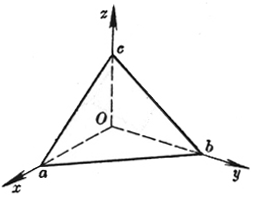a, b, c分别称为平面在三条坐标轴上的截距 [点法式]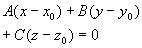(A, B, C不同时等于零)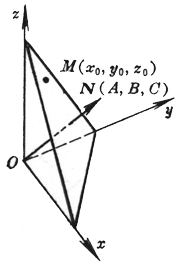平面通过点M(x0, y0, z0)，且法线N的方向数为A, B, C [三点式]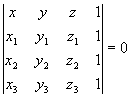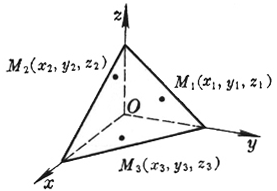平面通过三点: M1(x1, y1, z1) M2(x2, y2, z2) M3(x3, y3, z3) 或=0 方 程 与 图 形 说 明 [一般式] Ax +By +Cz +D = 0 (A, B, C为该平面的法线的方向数，且不同时等于零)当D=0时，平面通过原点 当A=0时(或B=0，或C=0)时，平面与x轴(或y轴，或z轴)平行 当A=B=0时(或A=C=0，或B=C=0)时，平面与Oxy平面(或Ozx，或Oyz)平行 [法线式]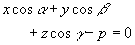(a , b , g 为平面的法线的方向角，p³ 0为法线长即原点到平面的距离)平面的一般式可化为法线式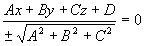，式中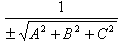称为平面的法化因子，当D< 0时取正号；D>0时取负号 [矢量式] (r -r0)× a = 0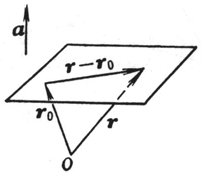平面 通过矢径r0的终点，且与已知矢量a垂直，r为平面上任意一点的矢径